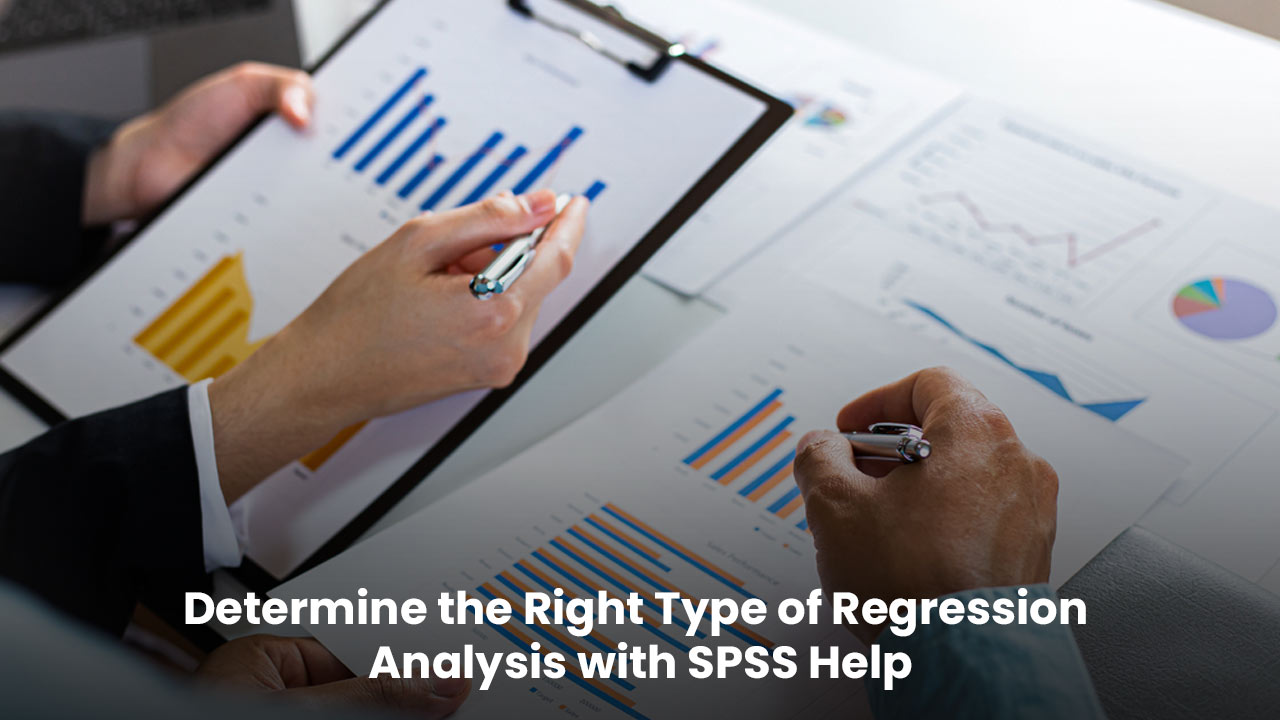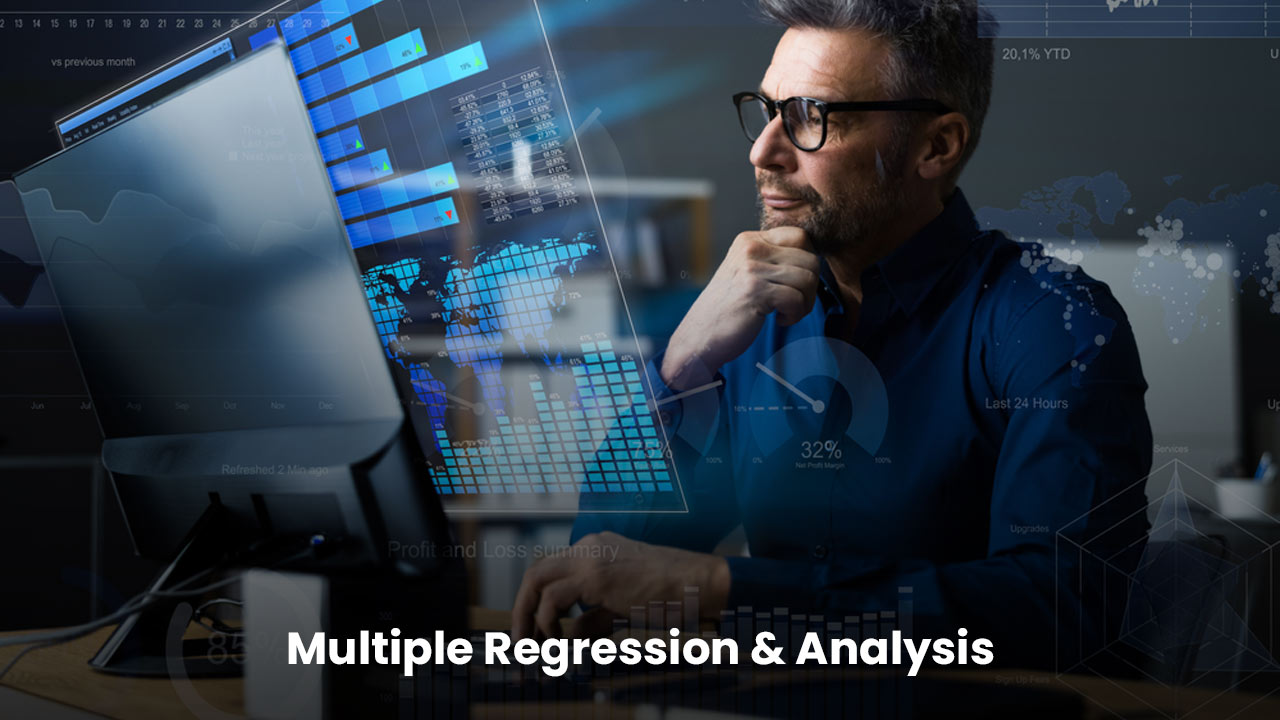# How to Determine the Right Type of Regression Analysis## Introduction

Regression analysis is utilised with SPSS help to define the relationship between a set of independent and dependent variables. Since regression analysis has several models to be used, it is hard to comprehend for students, which one to use with a particular set of variables. Basically, selecting the type of model depends on the kind of dependent variables you have, accordingly, the best-suited model is chosen.
This blog post will help you understand the different types of regression analyses with a better experience of the selection criteria of the right type. We will provide the entire information to choose the correct type. We will talk about the types of regression analysis models depending on the different types of dependent variables. When you are unsure of the procedure you are using, just know the type of dependent variable you have in your data and read the related section of this blog. This will help you narrow down your search for the correct choice. Let’s begin with the blog.

## Regression analysis using continuous dependent variables

This is the first type of regression analysis. Though this is the preliminary case, you still need clarification on which one to use.

1. ### Linear regression

Linear regression is also known as Ordinary Least Squares(OLS) or Linear Least Squares(LLS). It is the actual workhorse in the world of regression. This type of regression is used to witness the mean change in a dependent variable as per a one-unit change in each & every independent variable. Despite its term name, it can also use polynomials to model curvature and watch the effects of interaction. It minimises the sum of the squared errors, because of which such models are the most common and straightforward to use. If you are working with continuous dependent variables, then you should consider the linear regression model first. Linear regression has some special options available:

Fitted line plots: Use them when you have one independent and one dependent variable. These plots will display the data and regression outputs along the fitted line. These graphs make your model understanding more reflexive.

Stepwise regression: This is an automated method which can help you identify the candidate variables before the process of model specification.

Linear regression models were the first type of developed regression models so that the statisticians can do manual calculations. But OLS has a few lacunas, such as being sensitive to both outliers and multicollinearity and inclined to overfit. To overcome these limitations statisticians developed some advanced versions of OLS:

Ridge regression: You can use this advanced model even if high multicollinearity is present in the data. It, in fact, helps you prevent overfitting. It introduces a slight bias in the estimations to reduce the problematic variances caused by high multicollinearity. With this procedure, much of the variance is traded off for a little bias, resulting in more useful coefficient estimates when there is multicollinearity.

Lasso regression: It is equivalent to ridge regression but the only difference lies in its variable selection procedure. It selects variables to increase the prediction accuracy of the model by determining the simpler model.

Partial least square (PLS) regression: Use it when you have less number of observations as compared to independent variables or when you have highly correlated independent variables. First, PLS reduces the number of independent variables to uncorrelated components and then uses a linear regression model only on these reduced data components instead of the original data. PLS is not used for screening variables, but rather for developing predictive models. A continuous dependent variable can be included in a PLS model rather than an OLS model. In PLS, smaller effects are identified and multivariate patterns are modelled using the correlation structure in the dependent variables.

3. ### Non-linear regression

It also works with continuous dependent variables and is more flexible to fit curves than linear regression. It evaluates the parameters by minimising SSE. Unlike OLS, nonlinear regression models use matrix equations with iterative algorithms to solve problems. Thus, you must consider which algorithm to use, how to specify good starting values, and the possibility of a local minimum rather than a global minimum SSE if you do not converge on a solution.

## Regression analysis with categorical dependent variables

Depending on characteristics, categorical variables have values that can be categorised into several different groups. As opposed to least squares, the maximum likelihood estimation used in logistic regression transforms the dependent variable before estimating the independent variable. Logistic regression illustrates the relationship between independent variables and a categorical dependent variable. On the basis of the categorical dependent variable you have, you can choose the type of logistic regression model from the below-mentioned types:

1. ### Binary logistic regression

Binary logistic regression can be used to understand how changes in the probability of the occurrence of an event affect independent variables associated with it. As its name represents, a binary variable can have only two possible values such as pass or fail. Therefore, the binary logistic model can have binary dependent variables.

2. ### Ordinal logistic regression

Ordinal logistic regression defines the relationship between predictors and ordinal response variables. As an ordinal response, there are at least three groups that form a natural order, such as hot, medium, and cold.

3. ### Nominal logistic regression

It is also known as multinomial logistic regression. It determines the relationship between the independent variable and the nominal dependent variable. Nominal variables consist of at least three groups that do not form a natural sequence, such as scratch, dent, and tear.

## Regression analysis with count dependent variables

Use another type of regression model if your dependent variables are in the form of a number of items, events, activities, etc. These counts are always non-negative integers and high counts mean normal distribution for which you can use OLS. on the other hand, if your count data has a smaller mean then it can be skewed and linear regression will face difficulties fitting these data. In such cases, use one of the following regression models:

1. ### Poisson regression

Poisson regression is the best fit when using count data. Poisson variables are computations of something over a consistent length of observations. Using the Poisson variable you can estimate the rate of occurrence of a particular event. Poisson models are identical to logistic regression models because these models also work on Maximum Likelihood Estimation. These models recast the dependent variable using a natural log. If you are working with rate data types such as birthrate per month, then you should use the Poisson model.

2. ### Alternatives to the Poisson model

Because of some stringent restrictions, some count data do not work with the Poisson model. In such cases, you can use the following alternatives:

Negative binomial regression: The Poisson model only works when the variance is equal to the mean. But if the variance is greater than the mean, then your model will have overdispersion. In such a case negative binomial regression, also known as NB2 will be more suitable.

Zero-inflated models: Excessive zeros will find it difficult to follow the Poisson distribution, or we can say that the situation in which more zeros are present than the Poisson regression can predict, then this model won’t work here. Here, the zero-inflated model will be helpful. It is assumed that two independent processes work together to generate excessive zeros in zero-inflated models. One process checks if there are zero or more than zero events occurring, and on the other hand, Poisson processes how many events are occurring.

##Conclusion

These were different types of regression analysis models. If you are unable to figure out which method you should use for your data then you can take the help of this blog. You will definitely find a solution to your problem. But if you are still not sure about the type of model then you can take experts’ help for multiple regression analyses. Good luck!